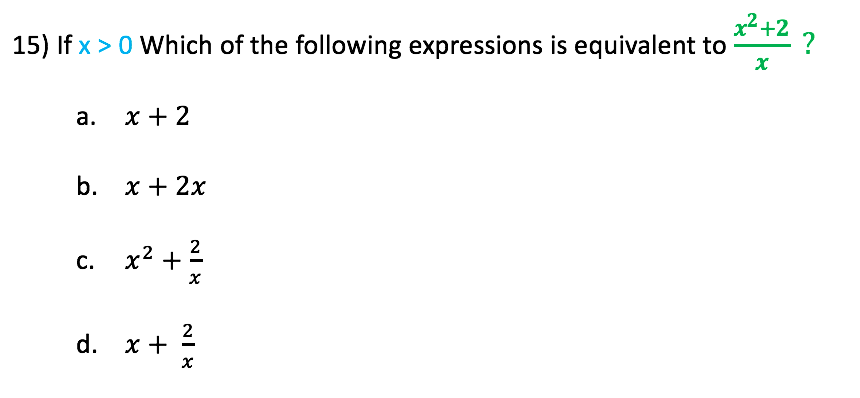top of page
Search

# Math: Picking Your Own Number

Updated: Mar 19, 2019Algebra. Some people love it…some don’t. One of the benefits of the SAT (and any other standardized multiple choice test) is that it doesn’t matter how you get the correct answer. The only thing that matters is that you do! For this reason, you can solve the math problem any way you like. Here at TutoringBoston, we suggest picking your own number for “x” whenever possible.

Let’s see how and why this works. Suppose the question was:Using our rule of exponents, we can see that the answer is “c.”

But suppose you don’t know how to work with exponents…don’t worry, you can still solve this math problem! Since the expression in the question must be equal to one of the answer choices, then the numerical value of the expression must be equal to the numerical value of the correct answer choice if you use the same number for “x” in both.

So, let’s pick a number for “x” and see how this plays out. Let’s suppose x = 3. We plug “3” into the original expression (to get 9), and into each of the answer choices, and see which answer choice also get us 9. We can see that the only one that matches is “c.”Now let’s practice this technique with another math problem.We can use “3” again. When we plug in x = 3 into the original expression, we get the number 11/3. So, when we plug x =3 into each of the answer choices, we know the correct answer is the one that also equals 11/3.When can I pick my own number:

You might have an opportunity to pick your own number when you see variables (“x,” “y,” “z” etc.) in the answer choices. There may be other ways to solve these types of problems - Tutoring Boston encourages students to know several approaches to problem solving so they have the option of deciding which technique is best.

How do I know which number to choose:

This is a great question because there are a few caveats to be aware of:

1) For most problems, avoid numbers such as “1” and “0” because they have unique properties. For example:

a. Anything multiplied by “1” is itself

b. Anything divided by “1” is itself

c. Anything multiplied by “0” is “0”

d. You can’t divide by “0”

2) For most problems, avoid complicated numbers such as fractions and decimals because they are harder to work with.

3) For specific types of problems, try these:

a. Problems involving percentages: try using “100”

b. Problems involving time: try using “60” or “120”

So, you want to pick numbers that are easy to manipulate, don’t have unique properties, and likely won’t generate more than one matching answer.

Let’s see what happens if for our first example, instead of x =3, we picked x = 1. The original expression equals “1,” so that is the number we are looking for…and it turns out that both “a” and “c” work.But since there can only be one single correct answer, we have to pick another number for “x” and try “a” and “c” again. As we know, if we pick x = 3, we see that only “c” works.

Interestingly, if we were to pick x = 2, we would get matching answers in both “b” and “c,” so we would have to pick another number and check "b" and "c". Again, as we know, if we pick x = 3, we see that only "c" works.Very important - make sure to eliminate 3 of the 4 answer choices:

Due to the nature of this technique, it is important to NOT stop until we have eliminated 3 of the 4 answer choices. As we saw above, it is possible for multiple answer choices to work, so we may have to plug in multiple times to eliminate false answers.

Picking your own number is a very powerful technique you can use on the math section on the SAT. When taking advantage of this strategy, please be conscious of the number you pick to avoid unnecessary work, and please make sure to eliminate 3 of the 4 choices - be ready to plug in another number if more than one answer choice works. Click here for more SAT tips and tricks.